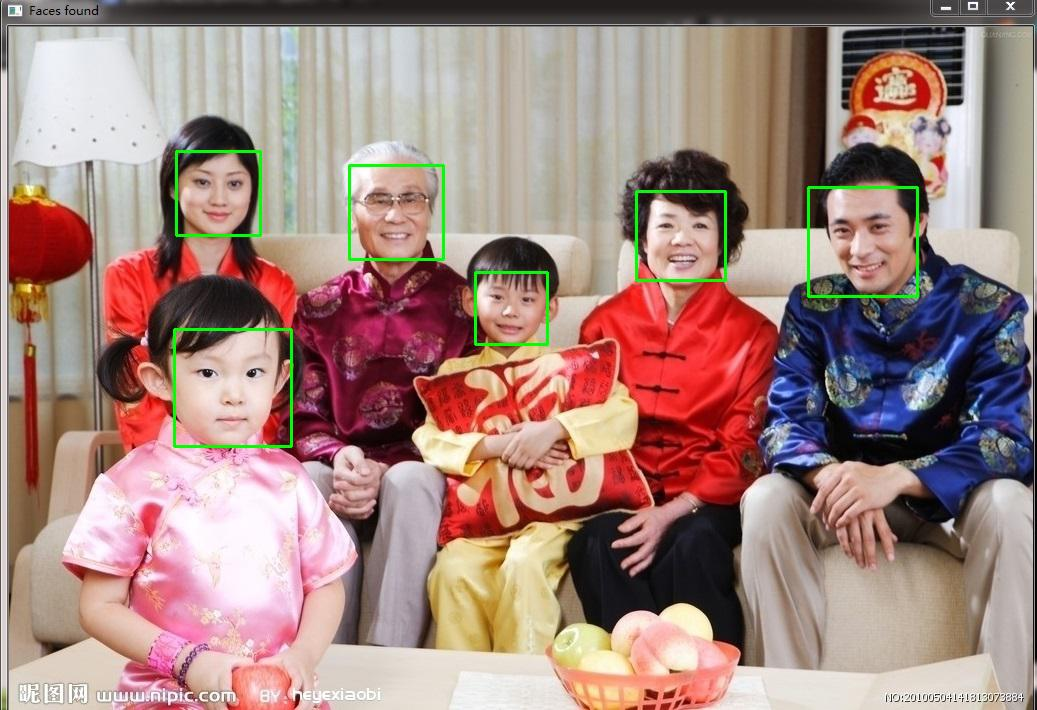import cv2
import sys
# Get user supplied values
imagePath = sys.argv
gray = cv2.cvtColor(image, cv2.COLOR_BGR2GRAY)#3
# Detect faces in the image
gray,
scaleFactor=1.15,
minNeighbors=5,
minSize=(5,5),
flags = cv2.cv.CV_HAAR_SCALE_IMAGE
) #4
print "Found {0} faces!".format(len(faces))#5
for (x, y, w, h) in faces:
cv2.rectangle(image, (x, y), (x+w, y+h), (0, 255, 0), 2) #6
cv2.imshow("Faces found", image)#7
cv2.waitKey(0) #8#1中

#2就是读取图像,

#3

gray = cv2.cvtColor(image, cv2.COLOR_BGR2GRAY)

#4

faces = faceCascade.detectMultiScale(
gray,
scaleFactor=1.15,
minNeighbors=5,
minSize=(5,5),
flags = cv2.cv.CV_HAAR_SCALE_IMAGE
)

gray : 进行检测的图像,这里是转换后的,

scaleFactor : 官网文档说是每次图片缩小的比例,其实可以这么理解,距离相机不同的距离,物体大小是不一样的,在物体大小不一致的情况下识别一个东西是不方便的,这就需要进行多次的缩放,这就是这个参数的作用.

minNeighbors : 可以理解为每次检测时,对检测点(Scale)周边多少有效点同时检测,因为可能选取的检测点大小不足而导致遗漏

minSize : 检测点的最小值,或者说就是检测点的最终值

flags : 这个参数在新版的opencv是不需要的,暂时不管了,注释掉同样可以

#5

<type ‘numpy.ndarray’>

#6

#7

<type ‘numpy.ndarray’>

#8